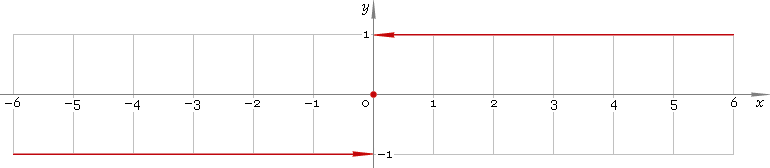The Art of Interface

# sign — signum function

Category. Mathematics.

Abstract. Signum: definition, plot and properties.

## 1. Definition

Signum function is defined as

sign(x) ≡ −1, for x < 0;
sign(0) ≡ 0;
sign(x) ≡ 1, for x > 0.

## 2. Graph

Signum function is defined everywhere on real axis — so, its domain is (−∞, +∞). Function plot is depicted below — fig. 1.Fig. 1. Plot of the signum function y = signx.

Function codomain is limited to the set of values {−1, 0, 1}.

## 3. Support

Signum function sign of the real argument is supported by free version of the Librow calculator.

Signum function sign of the complex argument is supported by professional version of the Librow calculator.

## 4. How to use

To calculate signum of the number:

``sign(-1.7);``

To calculate signum of the current result:

``sign(rslt);``

To calculate signum of the number x in memory:

``sign(mem[x]);``